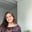Related Tags

frontend
html
web development
css

# How to maintain the aspect ratio of an image in HTMLUshna Ijaz

### Introduction

The aspect ratio of a screen or display is the proportional relationship between its width and height. An aspect ratio is represented by two integers separated by a colon which is the proportion between the width and height. In web design, we use the aspect ratio to describe that an image's width & height should be resized proportionally to each other.

### Maintain the aspect ratio

When we place an image on our webpage and resize it a little, we often run into the problem that the image goes out of proportion. There are a few ways to tackle this problem and preserve the aspect ratio of an image.

#### The object-fit property

In CSS, the object-fit property lets us adjust how we want our image to be displayed inside our image element. Let's look at how we can use this property.

The following code shows an image without applying the object-fit property. Click the run button to see the output. This might take a few seconds for it to appear.

Run the component below and notice how after applying the property, the image compresses and fits the width and height that we have set on the image tags.

To learn more about how to implement the object-fit property, click here.

#### The aspect-ratio CSS property

The aspect-ratio property allows us to maintain proportional dimensions where the height and width of an image are calculated automatically as a ratio.

#### Syntax

This property can take two values—auto and <ratio>. auto is the default value, whereas the <ratio> contains two numeric values separated by a / representing the width and height. We can see this here:

aspect-ratio: auto || <ratio>;

#### Examples of values for aspect-ratio

aspect-ratio: 4 / 3;
aspect-ratio: 16 / 9;
aspect-ratio: 1 / 1;
aspect-ratio: 0.5;


The following snippet demonstrates the implementation of the above-mentioned property:

#### Use automatic dimensions

The most common way to maintain the aspect ratio is to set the width and height of the image manually. We set the width of our image to our desired size and set the height to auto and vice versa. Here's how we do it:

<img src="image.jpg" style="width: 200px; height: auto;"/>
<img src="image.jpg" style="width: auto; height: 200px;"/>

RELATED TAGS

frontend
html
web development
css

CONTRIBUTORUshna Ijaz